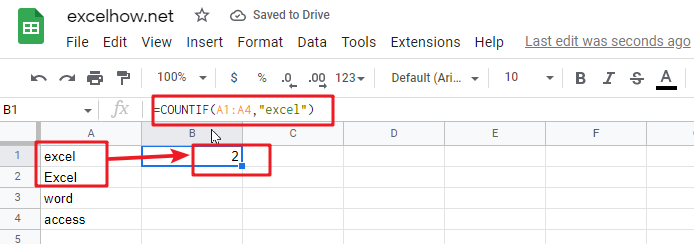# ExcelHow

This article will introduce you how to use the COUNTIF function in google sheets, and will show you a good example to better understand the usage of the COUNTIF function.

## Google Sheets COUNTIF Function Description

The google sheets COUNTIF function is used to count the number of cells in a cell range that meet a given condition. This function can be used to count cells which contain different types of values, such as numbers, dates, empty cells, non-empty cells or cells containing some special characters.

The COUNTIF function is a build-in function in Google Sheets and it is categorized as a Statistical Function.

You can use a logical operator in the COUNTIF function, such as: >,>=,<,<=<>,=, and the function also supports wildcards characters (*, ?) .

## Google Sheets COUNTIF Function Syntax

The syntax of the Google Sheets COUNTIF function is explained as follows:

`= COUNTIF (range, criteria)`

Where the arguments of the COUNTIF function are as follows:

• Range – This is a mandatory option, you want to use to apply the given conditions to count the number of matching cells in the specified cell range.
• Criteria – This is a mandatory option, it used to define which cells will be counted

## Google Sheets COUNTIF Function Examples

The below examples will show you how to use google sheets COUNTIF function to count the number of cells that match a given criteria in a cell range.

Example 1: If you want to count the number of cells that contain the “excel” text value in cell range B1:B4, then you can use the following COUNTIF formula：

`=COUNTIF(A1:A4,"excel")`You can see that the above COUNTIF formula returns a result of 2, which shows that B1: B4 range contains two “excel” text value of the cell.